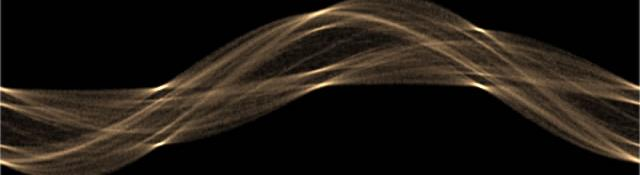## Hough transform in OpenCV

OpenCV already comes with a  function to perform hough transforms. It lets you choose between different variants of the transform: the standard hough transform, the probabilistic hough transform and the multi-scale hough transform. Here I'll get into the technical details of getting the command, `cvHoughLines2`, to work. The command expects and returns parameters in a certain format.

## The command

The cvHoughLines2 command goes like this:

```CvSeq* cvHoughLines2(CvArr* image, void* line_storage, int method, double rho, double theta, int threshold, double param1=0, double param2=0);
```

We'll go into each parameter in detail.

image is the image you want to do the hough transform on. This has to be an 8-bit single channel binary image. Though you can supply a grayscale image, it will be treated as a binary image (non-zero pixels are used).

line_storage is the place where this function stores its result. This can be either a CvMemoryStorage structure or a matrix with N rows. More on this parameter later.

method is either CV_HOUGH_STANDARD, CV_HOUGH_PROBABILISTIC, or CV_HOUGH_MULTI_SCALE. And you can guess they're for the standard hough transform, the probabilistic hough transform and the multi-scale hough transform.

rho and theta set the desired accuracy of the transform. rho is in pixels and theta is in radians. The smaller the value, the better the transform will be... but it'll also take more time. Usually, values 1 and 0.01 should be sufficient.

threshold determines which lines are returned. Each line has a particular number of "votes". This parameter sets the minimum number of "votes" in order to qualify as a potential line. You might want to read about The Hough Transform for more information on this.

param1 and param2 are used by the different transforms.

• For the standard hough transform, these are not used
• For the probabilistic hough transform, param1 is the minimum line segment length and param2 is the separation between collinear points to split them into two segments (instead of merging into a single one).
• For the multi-scale hough transform, rho/param1 and theta/param2 is the final resolution of the for refining results.

## Extracting results

Getting results out of this command depends on the line_storage parameter. You have two options: supply a CVMat matrix or supply a CvMemoryStorage stucture.

### The matrix approach

This one is straight forward. You give it a matrix, and the function will populate this matrix with its results. For different method values, this matrix must have different formats:

• Standard Hough transform and multi-scale hough transform: The matrix must be N rows by 1 column, and 2 channeled (`CV_32FC2`). It stores the p and θ values
• Probabilistic hough transform: The matrix must be N rows by 1 column, and 4 channeled (`CV_32FC4`). It stores the two end points of the line segments ( (x,y) twice). The function will set the number of rows of the matrix to the number of lines detected. Also, it will return a NULL.

### The CvMemoryStorage approach

If you provide a memory storage, the function will return a CvSeq* sequence. Using this sequence, you can access the various parameter of the detected lines:

```float* currentLine = (float*) cvGetSeqElem(line_seq , index);
```
• For the standard hough transform and the multi-scale hough transform, you can access the p and θ values using `currentLine` and `currentLine` (both float)
• For the probabilistic hough transform, the returned sequence is a sequence of CvPoint. So you can access the end points of line segments using `currentLine` and `currentLine` (both `CvPoint`)

## Done!

This should be enough to get you started with using the `cvHoughLines2` command!

#### Related posts#### Installing and configuring OpenCV 2.0 on Windows#### Installing and Getting OpenCV running#### OpenCV Memory Management

Utkarsh Sinha created AI Shack in 2010 and has since been working on computer vision and related fields. He is currently at Microsoft working on computer vision.